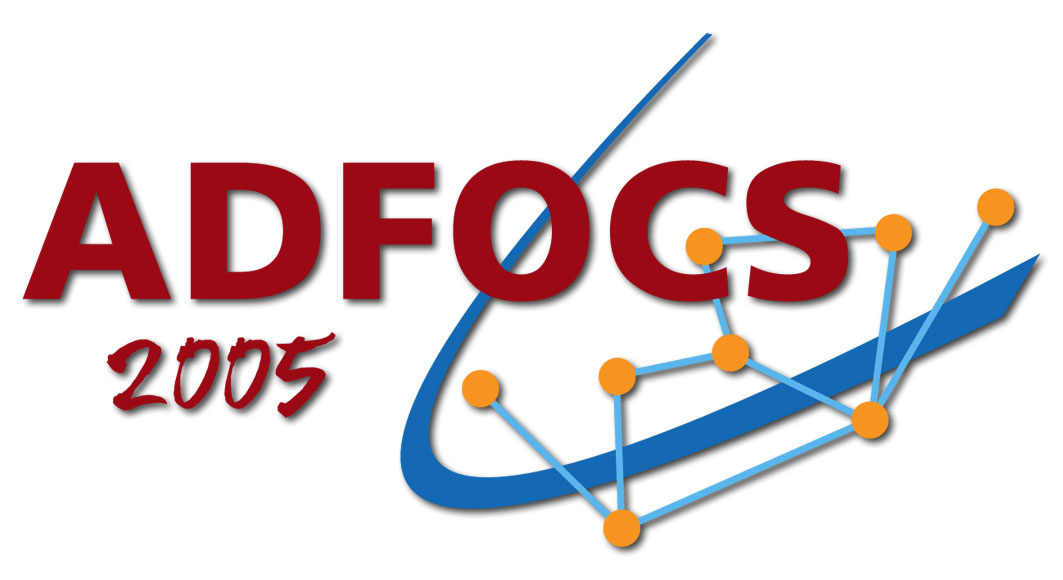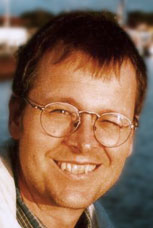6th Max-Planck Advanced Course on the Foundations of Computer Science August 29 - September 2, 2005 Saarbrücken, GermanyHOMEPAGE | PROGRAM | REGISTRATION | ORGANIZATIONAL NEWS | PICTURES

# Program

There will be eight blocks of lectures, exercises, and discussions, two per lecturer. Each block is about 4 hours. A morning or afternoon block will start with 1½ hours of lecture, followed by 1½ hours of exercises (in small groups) with coffee breaks, followed by a 1 hour discussion of the exercises. During the exercise periods, the respective lecturer will be around, as well as some fruit, snacks, and drinks.

## Schedule

August 29
Monday
August 30
Tuesday
August 31
Wednesday
September 1
Thursday
September 2
Friday
9.00-13.00 Erickson
Computing (with)
Curves on Surfaces I
Erickson
Computing (with)
Curves on Surfaces II
Rote
Pseudotriangulations I
Zwick
Approximating
Distances in Graphs I
Zwick
Approximating
Distances in Graphs II
13.00-14.30 Lunch
break
Lunch
break
Lunch
break
Lunch
break
Lunch
14.30-18.30 Klein
Dilation of Graphs
and Point Sets I
Klein
Dilation of Graphs
and Point Sets II
Excursion Rote
Pseudotriangulations II
Evening School Dinner

## Lecture(r)s

Below you find short abstracts and accompanying literature of the lectures. Clicking on the photos gets you to the respective lecturer's homepage.

## Jeff Erickson

University of Illinois at Urbana-Champaign

Computing (with) Curves on SurfacesThis tutorial will be a short and biased introduction to computational topology, focusing on algorithms that compute and/or use shortest paths and cycles on topological surfaces. For example, given a path around several obstacles in the plane, how quickly can we find the shortest path that misses the obstacles in exactly the same way? How quickly can we detect if one path on the Stanford Bunny can be continuously deformed into another? Given a surface, how quickly can we find the shortest cycle that cannot be collapsed to a point, or the shortest cycle that cuts the surface into two nontrivial pieces? The tutorial will include an introduction to basic concepts from topology, graph theory, and computational geometry; a survey of algorithms for computing shortest paths in different topological spaces; pointers to applications and more advanced results; and several open problems.

For preparation have a look at

1. Sergio Cabello, Yuanxin Liu, Andrea Mantler, and Jack Snoeyink,
"Testing homotopy for paths in the plane",
Discrete Comput. Geom. 31(1):61--81, 2004.
2. Jeff Erickson and Sariel Har-Peled,
"Optimally cutting a surface into a disk",
Discrete Comput. Geom. 31(1):37-59, 2004.
3. John Hershberger and Jack Snoeyink,
"Computing minimum length paths of a given homotopy class",
Comput. Geom. Theory Appl. 4(2):63--97, 1994.

## Rolf Klein

Universität Bonn

Dilation of Graphs and Point SetsOne of the basic problems in network design is the following. Given a set of points, find a network connecting the points such that the connecting paths are short and the cost of the network is low. This problem occurs in many variations. The network may be required to be part of a given graph; we may be allowed to use extra vertices; cost and connectivity can be measured in different ways.
In this lecture we will focus on points in the plane and on geometric networks N connecting them. If p and q are points of N then the dilation of p,q is defined as the ratio of the lenght of a shortest path in N that connects p and q, divided by their Euclidean distance. The maximum of these values, taken over all pairs of points from a certain subset S of N, is called the dilation of N with respect to S.
Well-known is the case where S equals the set of all vertices of N. Then the dilation of N is also called its stretch factor or its spanning ratio. But also the case where S consists of all points on N vertices and interior edge points alike, has received some recent interest under the name of detour or geometric dilation.
We shall first discuss some classical results on geometric spanners in the plane. Next, we will show how to efficiently compute the dilation of a given network, for some simple network structures. Then we turn to the following question. Given a finite set of points, S, what is the smallest possible dilation of a plane network that contains these points. This number is defined as the dilation of S. We present some results and many open questions.

For preparation have a look at D. Eppstein, "Spanning Trees and Spanners", Handbook of Computational Geometry

## Günter Rote

Freie Universität Berlin

PseudotriangulationsA pseudotriangulation is a decomposition of the plane into pseudotriangles, polygons with exactly three convex vertices and an arbitrary number of concave vertices. In the last years, pseudotriangulations have emerged as a data structure with a variety of applications: visibility complexes, ray shooting, robot arm motion planning, kinetic collision detection, guarding, reflex-free hulls, etc. I will survey some of the algorithmic, geometric and combinatorial properties of pseudotriangulations, as well as their connections to rigidity, convexity, and polytope theory.

For preparation have a look at

1. Günter Rote, Francisco Santos, and Ileana Streinu,
"Expansive motions and the polytope of pointed pseudo-triangulations",
Discrete and Computational Geometry - The Goodman-Pollack Festschrift. Editors: Boris Aronov, Saugata Basu, János Pach, and Micha Sharir, Algorithms and Combinatorics, 25, Springer Verlag, Berlin, 2003, pp. 699-736, arXiv:math.CO/0206027.
http://arxiv.org/abs/math.CO/0206027
http://page.mi.fu-berlin.de/~rote/Papers/abstract/
Expansive+motions+and+the+polytope+of+pointed+pseudo-triangulations.html
2. Ruth Haas, David Orden, Günter Rote, Francisco Santos, Brigitte Servatius, Herman Servatius, Diane Souvaine, Ileana Streinu, and Walter Whiteley
"Planar minimally rigid graphs and pseudo-triangulations",
Computational Geometry, Theory and Applications 31 (2005), 31-61, arXiv:math.CO/0307347.
http://arxiv.org/abs/math.CO/0307347
http://page.mi.fu-berlin.de/~rote/Papers/abstract/
Planar+minimally+rigid+graphs+and+pseudo-triangulations.html
3. David Orden, Günter Rote, Francisco Santos, Brigitte Servatius, Herman Servatius, and Walter Whiteley,
"Non-crossing frameworks with non-crossing reciprocals",
Discrete and Computational Geometry 32 (2004), 567-600, (Special issue in honor of Lou Billera), arXiv:math.MG/0309156
http://arxiv.org/abs/math.MG/0309156.
http://page.mi.fu-berlin.de/~rote/Papers/abstract/
Non-crossing+frameworks+with+non-crossing+reciprocals.html
.
Also available from Günter Rote's page.

## Uri Zwick

Tel Aviv University

Approximating Distances in GraphsComputing distances and shortest paths is one of the most basic algorithmic graph problems. Classical algorithms can be used to find all the distances in a graph with n vertices and m edges in about O(mn) time and O(n²) space. When the graph is very large these time and space bounds may be prohibitive. Can fewer resources be used if only approximate distance are required?
We present more efficient algorithms for computing approximate distances in undirected graphs. For unweighted graphs we get approximations with small additive error. For weighted graphs, with non-negative edge weights we get approximations with small multiplicative error.
We next consider the problem of finding sparse graphs, called spanners, that approximate well all the distances in the original graph. We obtain a tight trade off between the size of a spanner and its stretch and present efficient algorithms for finding good spanners.
We end with the construction of approximate distance oracles. These are algorithms for preprocessing a given input graph, using much less than O(mn) time and O(n²) space, such that each distance query can then be answered, approximately, in constant time.

For preparation have a look at

1. Dorit Dor, Shay Halperin, Uri Zwick,
"All Pairs Almost Shortest Paths",
SIAM Journal on Computing 29, 1740-1759 (2000).
2. Surender Baswana, Sandeep Sen,
"A Simple Linear time Algorithm for Computing (2k-1)-spanner of O(n^{1+1/k}) size for weighted graphs",
Proc. of ICALP'03, pp. 384-296.
3. Mikkel Thorup, Uri Zwick,
"Approximate Distance Oracles",
Journal of the ACM, 52, 1-24 (2005).
Draft and links to the first and the third paper are available from Uri Zwick's homepage . A draft of the Baswana-Sen paper is available on Surender Baswana's web page.